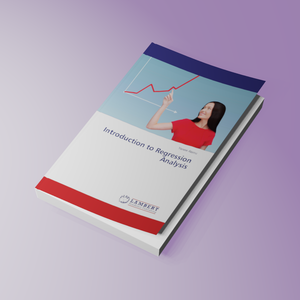Introduction to Regression Analysis

Regular price
€54,90
Sale price
€54,90
Regular price
Sold out
Unit price
per
Shipping calculated at checkout.

Summary:

Regression analysis deal with the relationship between dependent and independent variables while correlation analysis is concerned with measuring the strength of the relationship between two or more variables. This book explains in detail about simple and multiple linear regression, assumption of linear regression, model adequacy checking, methods of variable selection, transformation of data, binary logistic regression, count regression model (poisson, negative binomial, zero inflated poisson and zero inflated negative binomial regression model, model selection, parameter estimation and goodness of fit test).

Author:

Yenew Alemu

Biographie:

Yenew Alemu, Department of StatisticsCollege of natural and computational science, Injibara University, Injibara, Ethiopia

Number of Pages:

112

Book language:

English

Published On:

2019-07-30

ISBN:

978-3-659-63183-2

Publishing House: